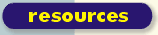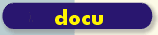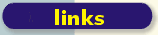scsh.net

# File To String List

Return a list of strings containing the lines of FILENAME.
```  (define (file->string-list filename)
(let ((port (open-input-file filename)))
(let lp ()
(let ((line-or-eof (read-line port)))
(if (eof-object? line-or-eof)
'()
(cons line-or-eof (lp)))))))

```

Tail-recursive version as suggested on comp.lang.scheme by John Gilson (http://groups.google.com/groups?dq=&hl=en&lr=&ie=UTF-8&selm=CQ3W9.39033%24yi6.6608966%40twister.nyc.rr.com)

```  (define (file->string-list filename)
(let ((port (open-input-file filename)))
(let lp ((all-lines '()))
(let ((line-or-eof (read-line port)))
(if (eof-object? line-or-eof)
(reverse! all-lines)
(lp (cons line-or-eof all-lines)))))))

```

Or, if you like CPS (Continuation-Passing Style):

```  (define (file->string-list filename)
(let ((port (open-input-file filename)))
(let lp ((k (lambda (x) x)))
(let ((line-or-eof (read-line port)))
(if (eof-object? line-or-eof)
(k '())
(lp (lambda (x) (k (cons line-or-eof x)))))))))

```

...though shouldn't these use WITH-INPUT-FROM-FILE instead of OPEN-INPUT-FILE, so there isn't an input port left hanging open?

Scheme is quite interesting but is there no easier way ? See Python for example:

print open('c:\config.sys','r').readlines()

or this way

lines = [line for line in open('c:\config.sys','r')]

That's just the convenience that a Python cursor (or, as they ambiguously name it, iterator) provides, which is actually not very convenient in complicated contexts (see Oleg's articles on cursors versus folding enumerators). It could easily be mimicked by using SRFI 40 streams instead of lists:

```  (define (port->line-stream in)
(let recur ()
(stream-delay
(let ((line (read-line in)))
(if (eof-object? line)
stream-null
(stream-cons line (recur)))))))

```

Then you can use SRFI 40's STREAM->LIST to convert the stream that (port->line-stream (open-input-file "wherever")) returns to a list, just as you do in Python with a list comprehension; you could also use any stream iteration function to use the lines, such as STREAM-FOLD or STREAM-MAP. (Note that there's no corresponding CLOSE-INPUT-PORT, and it's not written with CALL-WITH-OUTPUT-FILE; this has to do with the problems that cursors produce regarding resource management.)

Here's a much better, in general, way to operate on the sequence of lines from a port: using a folding enumerator:

```  (define (fold-port-lines kons in . knils)
(let ((knil-count (length knils)))
(let loop ((knils knils))
(let ((line (read-line in)))
(if (eof-object? line)
(apply values knils)
(receive new-knils (apply kons line knils)
(if (= (length new-knils) knil-count)
(loop new-knils)
(error "Too few knils"
`(expected ,knil-count)
`(got ,new-knils)))))))))

; This example of using it is almost as trivial as your Python version.
; The great difference is that we use CALL-WITH-INPUT-FILE instead of open().
; That automatically closes the port at the end.
(define (file->string-list fname)
(call-with-input-file fname
(lambda (in)
(fold-port-lines cons in '()))))

```

(This can trivially be converted into PORT->LINE-STREAM with CALL/CC or SHIFT & RESET; it also has no problems with resource management, so you could write a FOLD-FILE-LINES that uses CALL-WITH-INPUT-FILE instead of depending on the outside user to call CALL-WITH-INPUT-FILE and forcing them to use FOLD-PORT-LINES, because you know that the operation won't exit normally unless it has finished (a throw out of it may, of course, cause it to exit, but that's not 'normally,' and that is dealt with by either exiting normally by re-entering at some point, or the relevant continuation frames and the port proven by the garbage collector to be unreachable).)

BTW, there is port->string-list:

``` (port->string-list (open-input-file "foo.txt"))

```

---

Thank you very much for the detailed answer. I will first have to read Oleg's article(s) to which you have refered to get even started ;-)

FileToStringList - raw wiki source | code snippets archive